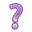## 10.5 Comparing between models

The goal: Find the subset of independent variables that optimizes (either minimize or maximize) a certain criteria. In other words, the goal is to find the optimal model.How do we measure “optimal”?

First we need to look at two quantities:

### 10.5.1 RSS: Residual Sum of Squares

Recall the method of least squares introduced in section 9 minimizes the residual sum of squares around the regression plane. This value is central to all following model comparison. How “far away” are the model estimates from the observed?

$\sum(Y - \bar{Y})^{2}(1-R^{2})$

### 10.5.2 General F Test

Two nested models are similar if the p-value for the General F-test is non-significant at a .15 level. Nested: The list of variables in one model is a subset of the list of variables from a bigger model. Similar to all other ANOVA models, you are essentially comparing the difference in RSS between nested models.

# Full model
full.employ.model <- lm(cesd ~ age + chronill + employ, data=depress)
# Reduced model
reduced.employ.model <- lm(cesd ~ age, data=depress)
anova(reduced.employ.model, full.employ.model)
## Analysis of Variance Table
##
## Model 1: cesd ~ age
## Model 2: cesd ~ age + chronill + employ
##   Res.Df   RSS Df Sum of Sq      F    Pr(>F)
## 1    292 22197
## 2    285 20036  7    2161.4 4.3921 0.0001197 ***
## ---
## Signif. codes:  0 '***' 0.001 '**' 0.01 '*' 0.05 '.' 0.1 ' ' 1
Caution: this uses anova() not aov().

Other references: https://online.stat.psu.edu/stat501/lesson/6/6.2

### 10.5.3 Likelihood function

What is the likelihood that we observed the data $$x$$, given parameter values $$\theta$$. $\mathcal{L}(\theta \mid x)=p_{\theta }(x)=P_{\theta }(X=x)$

• For strictly convenient mathematical matters, we tend to work with the log-likelihood (LL).
• Great because $$log$$ is a monotonic increasing function, maximizing the LL = maximizing the likelihood function.
• We can compare between models using functions based off the LL.

There are several measures we can use to compare between competing models.

### 10.5.4 Multiple $$R^{2}$$

If the model explains a large amount of variation in the outcome that’s good right? So we could consider using $$R^{2}$$ as a selection criteria and trying to find the model that maximizes this value.

• Problem: The multiple $$R^{2}$$ always increases as predictors are added to the model.
• Ex. 1: N = 100, P = 1, E($$R^{2}$$) = 0.01
• Ex. 2: N = 21, P = 10, E($$R^{2}$$) = 0.5
• Problem: $$R^{2} = 1-\frac{Model SS}{Total SS}$$ is biased: If population $$R^{2}$$ is really zero, then E($$R^{2}$$) = P/(N-1).

Reference PMA6 Figure 9.1

### 10.5.5 Adjusted $$R^{2}$$

To alleviate bias use Mean squares instead of SS.

$$R^{2} = 1-\frac{Model MS}{Total MS}$$

equivalently,

$$R^{2}_{adj} = R^{2} - \frac{p(1-R^{2})}{n-p-1}$$

Now Adjusted $$R^{2}$$ is approximately unbiased and won’t inflate as $$p$$ increases.

### 10.5.6 Mallows $$C_{p}$$

$C_{p} = (N-P-1)\left(\frac{RMSE}{\hat{\sigma}^{2}} -1 \right) + (P+1)$

where $$RMSE = \frac{RSS}{N-P-1}$$.

• Smaller is better
• When all variables are chosen, $$P+1$$ is at it’s maximum but the other part of $$C_{p}$$ is zero since $$RMSE$$==$$\hat{\sigma}^{2}$$

### 10.5.7 Akaike Information Criterion (AIC)

• A penalty is applied to the deviance that increases as the number of parameters $$p$$ increase.
• Tries to find a parsimonious model that is closer to the “truth”.
• Uses an information function, e.g., the likelihood function $$(LL)$$.

$AIC = -2LL + 2p$

• Smaller is better
• Can also be written as a function of the residual sum of squares (RSS) (in book)
• Estimates the information in one model relative to other models
• So if all models suck, your AIC will just tell you which one sucks less.
• Built in AIC() function in R
• Rule of thumb: Model 1 and Model 2 are considered to have significantly different fit if the difference in AIC values is greater than 2.

$\mid AIC_{1} - AIC_{2}\mid > 2$

### 10.5.8 Bayesian Information Criterion (BIC)

• Similar to AIC.
• Built in BIC() function in R
• Tries to find a parsimonious model that is more likely to be the “truth”. The smaller BIC, the better.

$BIC = -2LL + ln(N)*(P+1)$

### 10.5.9 AIC vs BIC

• Both are “penalized likelihood” functions
• Each = -2log likelihood + penalty
• AIC: penalty = 2, BIC: penalty = ln(N)
• For any N > 7, ln(N) > 2
• Thus, BIC penalizes larger models more heavily.
• They often agree.
• When they disagree, AIC chooses a larger model than BIC.